×

Search anything:

# Linear Probing in Hashing

#### Algorithms HashingGet this book -> Problems on Array: For Interviews and Competitive Programming

In this article, we have explored the algorithmic technique of Linear Probing in Hashing which is used to handle collisions in hashing. We have explained the idea with a detailed example and time and space complexity analysis.

### Table of content

1. Definitions and a little bit of history
2. Linear Probing
• Core Idea
• Insertion algorithm
• Searching algorithm
• Removal algorithm
• Time and Space Complexity
3. A way of implementation
4. Comparasion with other probing methods

## 1. Definitions and a little bit of history

Since the invention of the telephone by german professor Johann Philipp Reis in 1861, yes that is right, it seems it was not Graham Bell, people need a way to keep an evidence of their phone number agenda. To do so, they would have need for a name and a number associate with it, i.e. a key and a value, called in modern terms a hash table.

After aproximately a century, linear probing was invented in 1954 by Gene Amdahl, Elaine M. McGraw, and Arthur Samuel (and first analyzed in 1963 by Donald Knuth) , for resolving collisions in hash tables, and that happened long time before Dennis Ritchie,in 1972, at Bell Labs will invent the C langauge, that later on will be extended by Bjarne Stroustrup, still at Bell Labs, in 1985.

A hash table is a data structure that implements an associative array abstract data type, a structure that can map keys to values, for example a phone book.

A hash collision is when two keys in a hash table share the same value, for example two persons shares the same phone number. The probability of occurrance is given by the most common algorithms with varying levels of collision risk, CRC-32, MD5, and SHA-1. Since hash collisions are inevitable, hash tables have mechanisms of dealing with them, known as collision resolutions. Two of the most common strategies are open addressing and separate chaining.

## 2. Linear Probing

In this article we are going to refer at the Linear Probing which together with Double Hashing and Quadratic Probing forms the open addressing strategy.

• Core Idea

Cells in the hash table are assigned to one of the three states - occupied, empty, or deleted. If a hash collision occurs, the table will be probed to move the record to an alternate cell that is stated as empty.

• Insertion in Hash Table with Linear Probing
``````i <- hash(key)
loop
if array[i] is empty then
array[i] <- key
else
i <- (i + 1) mod size_of_array
end loop
``````
• Searching in Hash Table with Linear Probing
``````i <- hash(key)
loop
if array[i] = key or array[i] is empty then
return
else
i <- (i + 1) mod size_of_array
end loop
``````
• Removal in Hash Table with Linear Probing

After an element is removed, records in same cluster with a higher index than the removed one has to be recalculated. Otherwise the empty element left after deletion will cause valid searches to fail.

``````i <- hash(key)
if array[i] = key then
array[i] <- nil
for ( k<-i ; array[k] is not empty ; k++ )
j <- hash(array[k])
if i < j
array[i] <-> array[j]
``````

## Time and Space Complexity

For Insertion operation, the complexity analysis is as follows:

• Best Case Time Complexity: O(1)
• Worst Case Time Complexity: O(N). This happens when all elements have collided and we need to insert the last element by checking free space one by one.
• Average Case Time Complexity: O(1) for good hash function; O(N) for bad hash function

Assuming the hash function uniformly distributes the elements, then the average case time complexity will be constant O(1). In the case where hash function work poorly, then the average case time complexity will degrade to O(N) time complexity.

• Space Complexity: O(1) for Insertion operation

For Deletion operation, the complexity analysis is as follows:

• Best Case Time Complexity: O(1)
• Worst Case Time Complexity: O(N)
• Average Case Time Complexity: O(1) for good hash function; O(N) for bad hash function
• Space Complexity: O(1) for deletion operation

The ideas are similar to insertion operation.

Similarly for Search operation, the complexity analysis is as follows:

• Best Case Time Complexity: O(1)
• Worst Case Time Complexity: O(N)
• Average Case Time Complexity: O(1) for good hash function; O(N) for bad hash function
• Space Complexity: O(1) for search operation

## 3. A way of implementation

We can start by implementing a solution for a phone book case study.

In the first step we create a PhoneRecord class that stores information about the person name and the telephone number. These two can be associated with the key and value from our definitions. After that, we can declare as many individual persons, or objects, as we know but there will be no logic in addressing a person, each object is independent and does not affect the other ones as we might add for ex the "Pers 1" with two telephone numbers, which means the key will not be unique and that will result in a wrong addressing.

``````#include <iostream>
#include <string>

using namespace std;

class PhoneRecord
{
private:
string persName; //key
string telNo; // value

public :
PhoneRecord(string persName, string telNo)
{
if(this->persName != persName)
this->persName = persName;
if(this->telNo != telNo)
this->telNo = telNo;
}
void setRecord(string persName, string telNo)
{
if(this->persName != persName)
this->persName = persName;
if(this->telNo != telNo)
this->telNo = telNo;
}
string getPersName()
{
return persName;
}
string getTelNo()
{
return telNo;
}
};

int main()
{
PhoneRecord pr1("Pers 1", "tel_1");
PhoneRecord pr2("Pers 2", "tel_2");
PhoneRecord pr3("Pers 1", "tel_3");

cout<<pr1.getPersName()<<" "<<pr1.getTelNo()<<endl;
cout<<pr2.getPersName()<<" "<<pr2.getTelNo()<<endl;
cout<<pr3.getPersName()<<" "<<pr3.getTelNo()<<endl;

return 0;
}
``````

Output:

Pers 1 tel_1
Pers 2 tel_2
Pers 1 tel_3

To solve this issue, we'll add a new class named PhoneBook that has a finite dimension populated with an array of PhoneRecord objects and implementations of the insert, delete and search methods.

With the open addressing technique, a hash collision is resolved by probing, or searching through alternative locations in the array (the probe sequence) until either the target record is found, or an unused array slot is found, which indicates that there is no such key in the table.

The principle of this idea is to look at the hashKey implementation which should compute an index for the given key. Since our domain is a string and codomain is an int we must cast the string to int. The simplest way is to sum up each char of the string. But this will not solve our problem. For example "Pers 1" and "1 Pers" are made up by the same characters, and simply sum up the corresponding integer values will result in having the same value.

What we need to do next is to implement a computation for hashing strings, meaning to convert the string key into an unique number that is different regardless combinations of characters that compose it. And here are where collisions appear, given two different strings, the result of the hash key might be the same in some exceptional cases.

The pseudo code for this implementation is given by the next function:

``````    hash(String x, int a, int p)
h <- INITIAL_VALUE
for ( i <- 0 ; i < x.length ; i <-i+1 )
h <- ((h*a) + x[i]) mod p
return h
``````

where
* x represents our key
* INITIAL_VALUE represents a random number from a universal family mapping integer domain
* a represents the prime number multiplication
* p represents the dimension of our array

The next implementation will raise a collision since all slots are occupied.

``````#include <iostream>
#include <string>

using namespace std;

const int INITIAL_VALUE = 0;
const int PRIME_FACTOR = 31;

class PhoneRecord
{
private:
string persName; //key
string telNo; // value

public :
PhoneRecord(string persName, string telNo)
{
if(this->persName != persName)
this->persName = persName;
if(this->telNo != telNo)
this->telNo = telNo;
cout<<"One record inserted:"<<this->persName<<" "<<this->telNo<<endl;
}
void setRecord(string persName, string telNo)
{
if(this->persName != persName)
this->persName = persName;
if(this->telNo != telNo)
this->telNo = telNo;
cout<<"One record changed:"<<this->persName<<" "<<this->telNo<<endl;
}
string getPersName()
{
return persName;
}
string getTelNo()
{
return telNo;
}
};

class PhoneBook
{
private:
int dimension;
PhoneRecord **pr;

public:
PhoneBook(int dimension)
{
this->dimension = dimension;
pr = new PhoneRecord* [dimension];
}
int hashKey(string key)
{
int h = INITIAL_VALUE;

for (int i=0; i<key.size(); i++)

h = (h * PRIME_FACTOR + key[i]) % dimension ;

return h;
}
int find_slot(string key)
{
int i = hashKey(key) ;

if (pr[i] == NULL ) return i;
else
if (pr[i]->getPersName() == key) return i;
else
{
int j=0;
while(pr[i] != NULL && pr[i]->getPersName() != key && j < dimension)
{
i = (i+1) % dimension;
j++;
}
}
return i;
}
string lookup(string key)
{
int i = find_slot(key);

if (pr[i] != NULL && pr[i]->getPersName() == key)
return pr[i]->getTelNo();

return "no data found";
}
void set(string key, string value)
{
int i = find_slot(key);

if (pr[i] != NULL)
if (pr[i]->getPersName() == key)
pr[i]->setRecord(key, value); // change the value of the occupied key
else cout << "collision";

else pr[i] = new PhoneRecord(key, value); // alocate memory for the unoccupied slot
}
void remove(string key)
{
int i = find_slot(key);
if ( pr[i] != NULL && pr[i]->getPersName() == key)
{
pr[i] = NULL;
cout<<"One record removed:"<<key<<endl;
}
else
cout<<"Nothing to remove"<<endl;
}
};

int main()
{
PhoneBook pb(3);

pb.set("Pers 1","Tel 1");

pb.set("Pers 2","Tel 1");

pb.set("Pers 3","Tel 3");

pb.remove("Pers 2");

pb.set("Pers 4","Tel 4");

pb.set("Pers 4","Tel 2");

cout<<pb.lookup("Pers 2")<<endl;

pb.remove("Pers 1");

pb.set("Pers 5","Tel 1");

pb.remove("Pers 1");

pb.remove("Pers 3");

pb.set("Pers 6","Tel 6");

pb.set("Pers 7","Tel 7");

return 0;
}
``````

Output:

One record inserted:Pers 1 Tel 1
One record inserted:Pers 2 Tel 1
One record inserted:Pers 3 Tel 3
One record removed:Pers 2
One record inserted:Pers 4 Tel 4
One record changed:Pers 4 Tel 2
no data found
One record removed:Pers 1
One record inserted:Pers 5 Tel 1
Nothing to remove
One record removed:Pers 3
One record inserted:Pers 6 Tel 6
collision

## Step by step example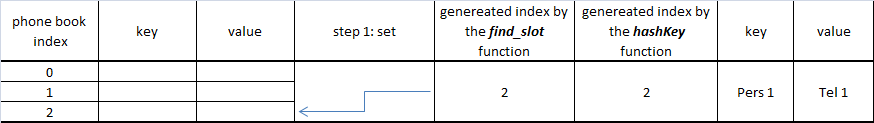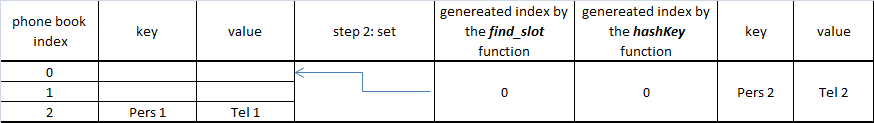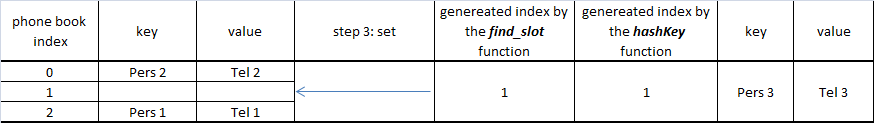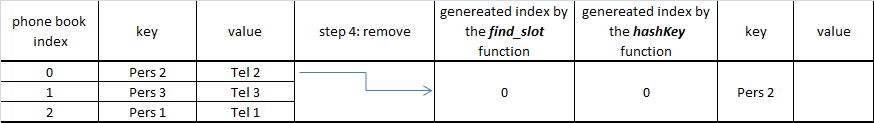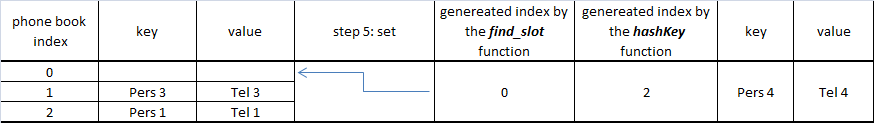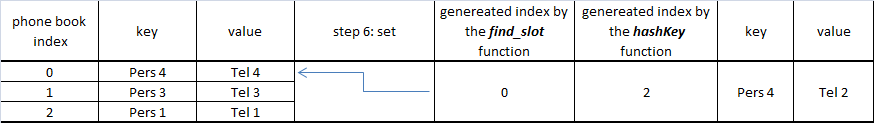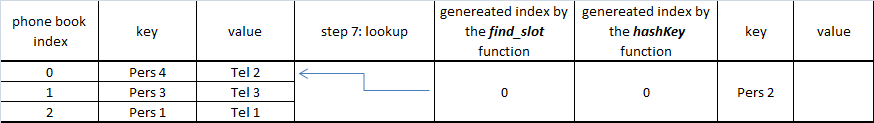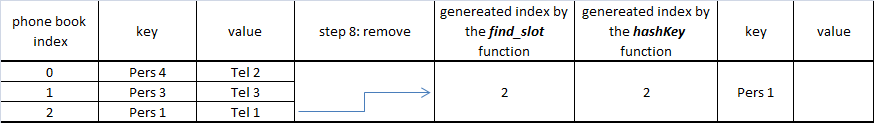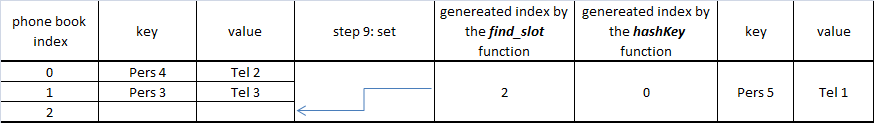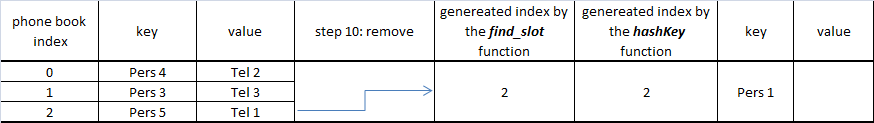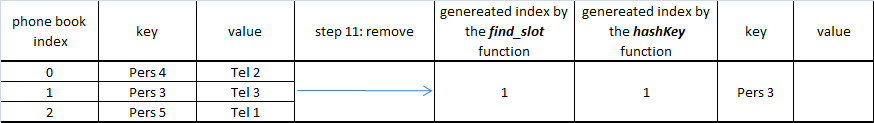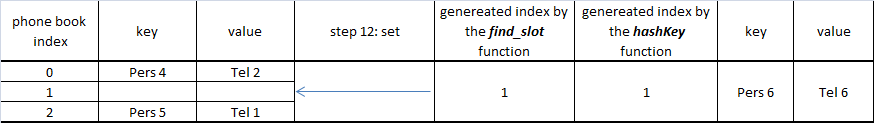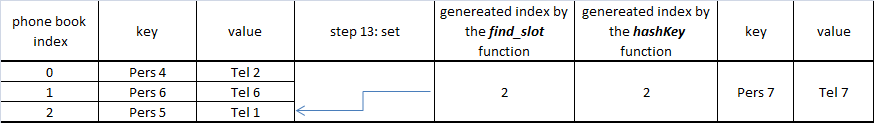At the final step "Pers 7" does not have an empty slot, so collision appears with the "Pers 5" which will share the same cell.

In this situation, there are two posibilities:

1. replace the existing key with the new one
2. increase dimension of the table, usually this is recommended to be done when table is 80% full of capacity, and realocate all the existing keys to the new table.

## 4. Comparasion with other probing methods

Linear probing - the interval between probes is fixed — often set to 1.

Quadratic probing - the interval between probes increases quadratically (hence, the indices are described by a quadratic function).

Double hashing - the interval between probes is fixed for each record but is computed by another hash function.

Some open addressing methods, such as Hopscotch hashing, Robin Hood hashing, last-come-first-served hashing and cuckoo hashing move existing keys around in the array to make room for the new key. This gives better maximum search times than the methods based on probing.

With this article at OpenGenus, you must have the complete idea of Linear Probing in Hashing.